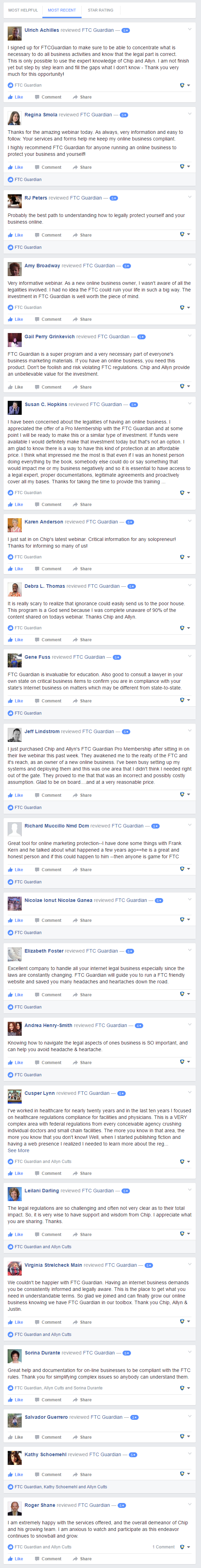``` (function (v, i, d, a, l, y, t, c, s) { y='_'+d.toLowerCase();c=d+'L';if(!v[d]){v[d]={};}if(!v[c]){v[c]={};}if(!v[y]){v[y]={};}var vl='Loader',vli=v[y][vl],vsl=v[c][vl + 'Script'],vlf=v[c][vl + 'Loaded'],ve='Embed'; if (!vsl){vsl=function(u,cb){ if(t){cb();return;}s=i.createElement("script");s.type="text/javascript";s.async=1;s.src=u; if(s.readyState){s.onreadystatechange=function(){if(s.readyState==="loaded"||s.readyState=="complete"){s.onreadystatechange=null;vlf=1;cb();}};}else{s.onload=function(){vlf=1;cb();};} i.getElementsByTagName("head").appendChild(s); };} vsl(l+'loader.min.js',function(){if(!vli){var vlc=v[c][vl];vli=new vlc();}vli.loadScript(l+'player.min.js',function(){var vec=v[d][ve];t=new vec();t.run(a);});}); })(window, document, 'Vidalytics', 'vidalytics_embed_sLhwRISRAXrI203H', 'https://fast.vidalytics.com/embeds/INqGgqKH/sLhwRISRAXrI203H/'); ```